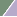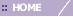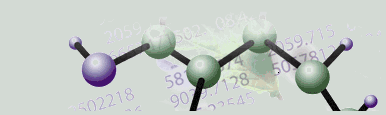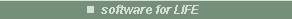# Jarvis-Patrick Clustering Overview

Overview

Jarvis-Patrick clustering is a clustering method based on similarity between neighbors. Similarity (or closeness) is determined by using a distance metric. One or more Neighbors in Common are used to judge the cluster membership of the objects under study. The function is deterministic and non-iterative.

Algorithm Properties

• The algorithm chooses the number of clusters.

• There is always at least one item in each cluster.

• The algorithm partitions the input into non-hierarchical clusters.

• The clusters do not overlap.

• If two different items from the input dataset share enough mutual nearest neighbors, then those two items are in the same cluster.

Parameters

General clustering parameters, distance measurements between data points, and distance measurements between clusters are used to perform this procedure. In addition to these general clustering parameters, there are two parameters specific to the Jarvis-Patrick algorithm:

• the number of Neighbors to Examine

• the minimum required number of Neighbors in Common.

The first parameter, Neighbors to Examine, specifies how many of each item's neighbors to consider when counting the number of mutual neighbors shared with another item. This value must be at least 2. Lower values cause the algorithm to finish faster, but the final set of clusters will have many small clusters. Higher values cause the algorithm to take longer to finish, but may result in fewer clusters and clusters that form longer chains.

The second parameter, Neighbors in Common, specifies the minimum number of mutual nearest neighbors two items must have for them to be in the same cluster. This value must be at least 1, and must not exceed the value of the Neighbors to Examine parameter. Lower values result in clusters that are compact. Higher values result in clusters that are more dispersed.

Basic Procedure

• For each object, find its J-nearest neighbors where ‘J’ corresponds to the Neighbors to Examine parameter on the Partitional Clustering dialog.

• Two items cluster together if they are in each other’s list of J-nearest neighbors and K of their J-nearest neighbors are in common, where the K value corresponds to the Neighbors in Common parameter on the Partitional Clustering dialog.

In GeneLinker™, input provided to the algorithm is as follows:

• The dataset.

• A distance metric.

• The number of nearest Neighbors to Examine.

• The number of nearest neighbors two data points must share to be in the same cluster (Neighbors in Common).

When to Use The Jarvis-Patrick Algorithm

Use this algorithm when you need to work with non-globular clusters, when tight clusters might be discovered in larger loose clusters, when a deterministic partitional clustering result is desired, or when clustering speed is an issue since the algorithm is not iterative.

Related Topics:

Tutorial 3: Jarvis-Patrick Clustering